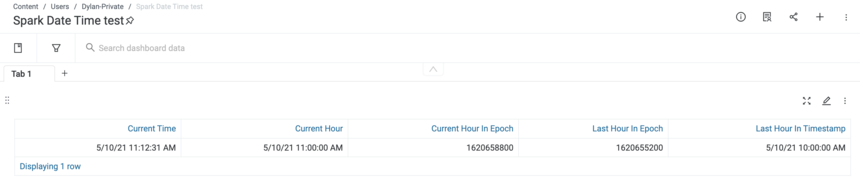0

How to handle Date Time in Spark MV

We would like to get the current hour, how can we get it in Spark SQL?   We also need the time as of one hour ago, how do we do that?

Here is a sample code that show how we can produce it using Spark.  There are more than one way to do it.

We can use epoch time as the bridge for doing many calculations.

df = spark.sql('''
SELECT  current_timestamp() CURRENT_TIME,
DATE_TRUNC('HOUR',current_timestamp()) CURRENT_HOUR,
CAST(DATE_TRUNC('HOUR',current_timestamp()) AS LONG) CURRENT_HOUR_IN_EPOCH,
CAST(DATE_TRUNC('HOUR',current_timestamp()) AS LONG) - 60*60 LAST_HOUR_IN_EPOCH,
timestamp(CAST(DATE_TRUNC('HOUR',current_timestamp()) AS LONG) - 60*60 ) LAST_HOUR_IN_TIMESTAMP
''')

save(df)

It shows results: